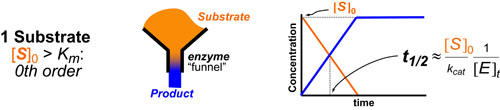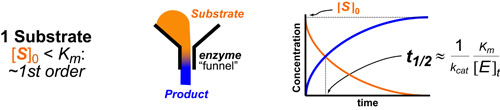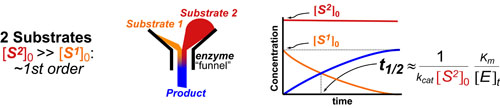# Kinetics #1: Catalyzed Reaction TimescalesMost chemical and biochemical research concerns reaction that are catalyzed by either an enzyme or a chemical catalyst. Unfortunately, when we learn chemical kinetics, it is usually in the context of idealized: uncatalyzed 0th-order, 1st-order of 2nd-order chemical reactions. Luckily the intuition we learn from 0 and 1st order processes can be readily extended to catalyzed processes by invoking the pseuso-1st order approximation. Below we describe how to “think about” the most common enzymatic/catalytic kinetic curves you may encounter.

Here, we graphically represent a catalyzed chemical reaction using: a funnel to represent the enzyme/catalyst(E), orange or red to represent Substrate(S) and blue to represent product(P). Otherwise this post uses similar convensions as our post on the Michaelis-Menton Equation where Michaelis-constant (Km) describes how tightly the substrate binds the enzyme/catalyst, kcat is a rate-constant that describes how quickly the enzyme can make the product, and kobs represents the pseudo-1st order rate constant. Brackets denote concentrations where: a 0 subscript represents “initial concentration”, a t subscript represents “total concentration.” t1/2 represents the timescale or approximate half-life of substrate decay or product formation. The curves represented here are kinetic curves that plot time (x-axis) versus concentration (y-axis).

In the first common scenario, if your enzyme/catalyst is saturated, the rate of the process is 0th order (i.e. a constant(Vmax)) then your kinetic curves will exhibit a linear shape. This occurs when the inital concentration of substrate is much higher than the Michaelis-Constant (or [S]0 >> Km see previous post on Michaelis-Menton Equation).In the second common scenario, if you enzyme/catalys is not saturated, the rate of the process is pseudo-1st order the curves exhibit exponential shapes. Under pseudo-1st order conditions the timescale/half-life (t1/2) of the process can be well approximated by 1/kobs which is explicitely defined in the figure below.In the third common scenario, if you enzyme/catalys is not saturated and you have two substrates, the rate of the process can still be considered pseudo-1st order if you assume that the concentration of one substrate (S2) is much higher than the other (S1). Under pseudo-1st order conditions the timescale/half-life (t1/2) of the process can be well approximated by 1/kobs which is explicitely defined in the figure below.

### REFERENCES:

1. Segel, I.H. Enzyme Kinetics: Behavior and Analysis of Rapid Equilibrium and Steady-State Enzyme Systems, Wiley-Interscience, 1993
2. Banford, C.H.; Tipper, C.F.H. Comprehensive Chemical Kinetics. Elsevier, 1969
3. McBane, G.C. Graduate Chemical Kinetics Lecture Notes. OSU875, http://faculty.gvsu.edu/mcbaneg/chem875.pdf
4. Lauffenburger, D.A. Receptors: Models for Binding, Trafficking and Signalling, Oxford University Press 1993.